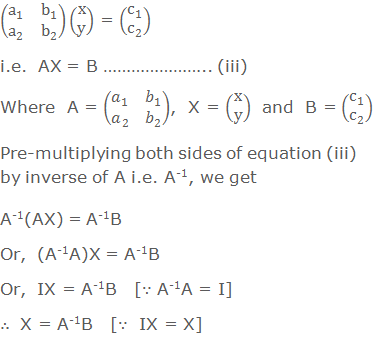# Solving Equations by Matrix Method## Solving Equations by Matrix Method

The simultaneous equations in two variables can be solved by different methods: substitution method, cross multiplication method, etc. Here, we deal with the method of solving linear equations of two variables using matrices.

********************

10 Math Problems officially announces the release of Quick Math Solver, an Android App on the Google Play Store for students around the world.

********************

Let us consider the following linear equations,

a1x + b1y = c1 ………………. (i)

a2x + b2y = c2 ………………. (ii)

Writing equations (i) and (ii) in the matrix form, we getEquating the corresponding elements of equal matrices X and A-1B, we get the solution.Note: If the determinant |A| = 0, the system of simultaneous equations have no unique solution, therefore when |A| ≠ 0, the equations have unique solution.

### Worked Out ExamplesExample 3: If the cost of 17 kg sugar and 4 kg tea is Rs. 1110 and the cost of 8 kg sugar and 2 kg tea is Rs. 540. Find the cost of sugar per kg and tea per kg by matrix method.

Solution: Let,

Cost of sugar per kg = Rs. x

Cost of tea per kg = Rs. y

Then according to questions,

1st case, 17x + 4y = 1110 …………… (i)

2nd case, 8x + 2y = 540 ……………… (ii)

Writing the given equations in the matrix form, we getComparing the corresponding elements, we get

x = 30, y = 150

Hence, The cost of sugar per kg = Rs. 30

The cost of tea per kg = Rs. 150

Do you have any questions regarding the matrix method for solving equations?

You can ask your questions or problems here, in the comment section below.